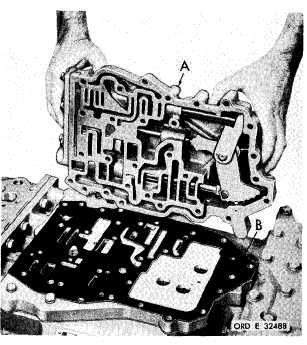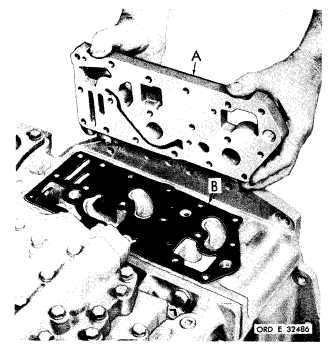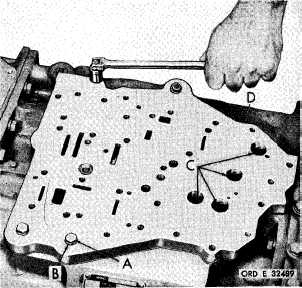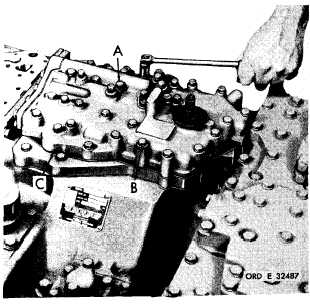Custom SearchP A R   5    S T E P S   3 - 6 D I S A S S E M B L Y C H A P   5,   SEC   II Figure   67   (Step   3) R e m o v e    r e g u l a t o r    a n d    l o c k u p    s h i f t    c o n t r ol valve   body   oil   transfer   plate   (A)   and   gasket   (B). Figure   68   (Step   4) Using   a   l/2-inch   wrench,   remove   28   bolts   (A) a n d    l o c k    w a s h e r s    ( B )    r e t a i n i n g    m a i n    c o n t r ol valve   body   assembly   (C). Figure   69   (Step   5) Remove   main   control   valve   body   assembly   (A) and   gasket   (B). Figure   70   (Step   6) U s i n g    a    l / 2 - i n c h    w r e n c h ,    r e m o v e    f o u r    b o l ts (A)   and   lock   washers   (B)   and   four   self-locking bolts   (C)   with   flat   washers   retaining   main   con- trol   valve   body   oil   transfer   plate   assembly   (D). 8 5